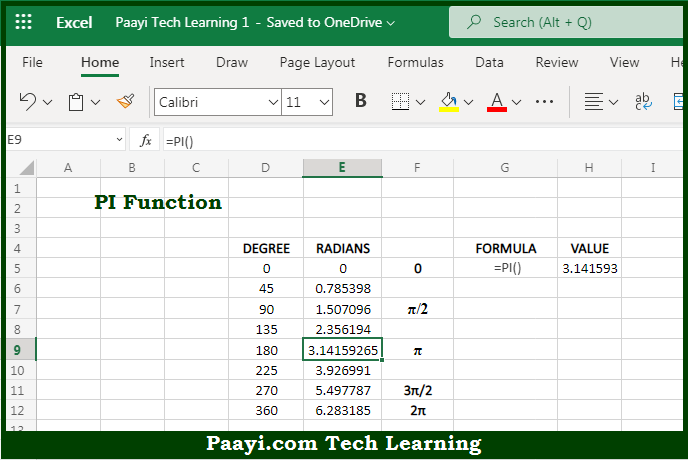# Learn How to Use Microsoft Excel PI Function

Written by | 0 Comments | 677 Views

In this article, you will learn how to use the Microsoft Excel PI function and its prime function in Microsoft Excel. You will also get to know the Microsoft Excel PI function return value and syntax with the help of some examples.

Microsoft Excel PI Function

The main function of the Microsoft Excel PI function is to get the value of π. That implies, with the help of the PI function you can able to return the value of the geometric constant π or pi. The value represents the half-rotation in the radian angle system. You must have observed that this constant appears in many formulas which are related to circle. So, with the help of the PI function, you can able to get the value of pi.

Return Value of PI Function

The return value will be 3.14159265358979.

Syntax of PI Function

=PI( )

## How to Use Microsoft Excel PI Function?So we know that Microsoft Excel PI function you can able to get the value of π. That implies, with the help of the PI function you can able to return the value of the geometric constant π or pi. The value represents the half-rotation in the radian angle system. You must have observed that this constant appears in many formulas which are related to circle. So, with the help of the PI function, you can able to get the value of pi.